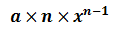top of page

# Absolute and local minimum and maximum using derivatives (Can we use UEFA records?)

In this blog, we will discuss how to find the absolute and local extrema using derivatives because we are not going to be working with graphs (Take a deep breadth Donald Trump and say 'easy easy' because it is easy).

Can we use UEFA league records as example?It depends on what values we are using. This is because the first rule to use derivatives to find the extrema is that the function should be continuous. This means the graph should not break in between. The goals scored by top scorers such as Cristiano Ronaldo and Lionel Messi by season are not continuous as one cannot obtain results in years 1.2 or 1.5 or any similar random point.

However, we could use the number of spectators that entered the stadium during a match against time.Let's get started with absolute maximum

Let's assume the number of spectators that gathered to watch a EURO match on a particular day form a polynomial equation where t is the number of minutes:This has to be evaluated on the closed interval's end points [0, 90 ) where the total time of the match was 90 minutes.

We need to first find the critical point. What is this point? This is where the slope of the curve is zero. If it was a straight line in the form of y=mx+c we know the slope is m. But what is the slope of a curve with a polynomial function? The slope is the derivative of the given function. Hence, in order to find the derivative or the slope of our given function f(t), we have to differentiate f(t).How do we differentiate?

Well, Donald Trump, this is not rocket science. Any given expression in the form ofis differentiated as follows:Now we have to equate the differentiated function to zero. This is because we have now found the slope and the critical points appear where the slope is zero.Solving the above equation gives us the following results:We know that 54 is within the given interval but not -2. So we will not be evaluating the function at t=-2. However, we need to evaluate the function at the end points t=0 and t=90, in addition to the critical point we found (i.e. t=54).

Now Donald Trump, its all about substituting the t values in f(t) to find in which minute of the game was the stadium filled with the highest spectators and what was that number.Hence, the stadium was at an absolute maximum at the 90th minute of the game. Hold on Donald. 90 is not the absolute maximum. The absolute maximum is the result which is 101600 spectators.

Moving on to absolute minimum

This is now easy because it is found using the same technique we used to evaluate absolute maximum. We found above, that the lowest amount of spectators was at the 54th minute of the match which is 49760. Hence, 49760 is the absolute minimum number of spectators during the match.

Local minimum and maximum

As we discussed in the previous blog, the local extrema are evaluated the same way with the exception that we use only the given interval. For instance, if we choose a domain between the 0th and 60th minute, then we have to substitute t=0, t=60 and t=54 (the critical point) to f(t) and find the answer.Now, the local maximum is 78920 spectators at the 0th minute (the time just before the match started) and the local minimum is 49760 spectators (the 54th minute).

Hope you understood Donald. See you soon with another interesting topic.